1671252320

## How to Build a Your Own Wordle For Numbers: Numble

After Wordle overtook the world and my Twitter feed, I, like the rest of the world, became somewhat obsessed. I became so obsessed that I was struck with the idea of making an app, similar but with a focus on numbers. Two weeks later, Numble was born — a Wordle for numbers.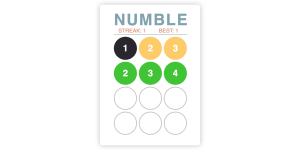## The Rules of Numble

It really is quite simple: guess the three-digit multiple of three.

You have four guesses, and after each guess the colors of each digit change depending its position and whether it’s actually in the Numble.

• Green: the digit is in the right place.
• Yellow: the digit is in the Numble but in the wrong place.
• Grey: the digit isn’t in the Numble at all.

If you’ve forgotten from school, the rule for knowing whether a number is a multiple of three, is if the digits add up to a multiple of three.

For example: 123 is a multiple of three because 1 + 2 + 3 = 6.

## Nanny State

To make building Numble easier, I used a small library called Nanny State. It’s written by Darren Jones, in case you missed it he wrote an article introducing it recently. It stores all the app data in a single object called State and then re-renders the HTML view automatically depending on any changes made to the State. For it’s speed and efficiancy and no new syntax, it’s remarkably simple and easy to learn.

First things first, we need to import Nanny State and set up `State``View` and `Update`.

To import it you just need to copy this line of code into the very first line of your program:

``````import { Nanny,html } from 'https://cdn.skypack.dev/nanny-state'
``````

If you want to learn a little bit more about what you’re importing and how the structure of everything is working, make sure to check out the link above. Next, we need to create the View:

``````const View = state => html`
<h1>Numble</h1>`
``````

The `View` is a function that returns a string that is basically the HTML that will be displayed on our page. This is a basic layout to get us started and once everything is in place, a heading of ‘Numble’ should appear. It takes `State` as a parameter, giving us access to the data stored in the single object.

Now we need to create the State object, and this is where any data will be stored, but for now the only porperty it needs is `View`, linking them together.

``````const State = {
View
}
``````

And finally, we need to call the `Nanny` function. This will render the initial view. We also assign the variable `Update` to the return value. This will allow us to update the state later on.

``````const Update = Nanny(State)
``````

Your page should look like this:Here’s what the code should look like altogether:

Now Nanny State is set up, we can start making the game, which by the end should be a fully functioning ‘Guess the Number’ game.

## Every End has a Start

A few things before we start, one of the benefits of the `View` function is that it uses template literals, which means we can add logic within the HTML itself. This comes in very handy when you want to include different views based on the State. Unfortunately, a standard `if` Statement won’t work and instead we will need to use ternary operators.

If you aren’t familiar already, ternary operators work in the same way as an if else statement: condition ? what to do if true : what to do if false. In short, trade the `if` for a `?` and the `else` for a `:`.

For example, here’s a great one I saw on Twitter the other day:

``````const toDo = amIHungry ? "🍰" : "😴"
``````

This is equivalent to:

``````if (amIHungry){
const toDo = "🍰"
}
else{
const toDo = "😴"
}
``````

Ternary operators are about to become your best friend so it’s pretty vital that you understand how they work.

A start button is a great way to add some structure to a game, especially one like Numble, and so in order to do this we’ll need to add a property to `State``started`. The value of `started` needs to be `false`, as the first thing we want the user to see is the menu page (which, for now, will consist of the start button and the heading).

`State` should now look like this:

``````const State = {
started: false,
start, finish,
View
}
``````

The `View` is what changes the most here and we can use our first and main ternary operator.

Notice that it includes two variables called `start` and `finish`. These are references to the event handlers that we will be writing soon.

In Nanny State, any event handlers need to be referenced inside the `State` object.

We basically want two different views: one for when the user has started (in other words, the game itself) and one for when the user hasn’t started (a menu page, perhaps). So we can use our Boolean property of `started` to do this.

``````const View = state => html`
<h1>Numble</h1>
\${state.started ?
html`<button onclick=\${state.finish}>END</button>`
:
html`<button onclick=\${state.start}>START</button>`
}`
``````

As you can see, the heading is above the ternary operator – this means it will remain visible for both pages of the program. So for the ternary operator, you might be able to see exactly what it’s doing already, but if not, don’t worry, it’s very simple.

It follows exactly the same logic as the cake/hungry example above: it checks if `state.started` is `true`. If it is, a button called ‘End’ is displayed. If not, a button called ‘Start’ is displayed instead.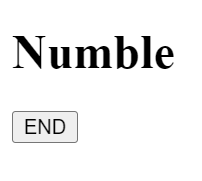The buttons themselves have inline event listeners: the ‘Start’ button has one that calls the function `start` and the ‘End’ button has one that calls the function `finish`. Obviously, these functions don’t exist yet, which leads us on to our next job: creating the event handlers.

We’ll do the `start` function first. It’s very simple, as all we need to do is update the `started` property to `true`. The code should look like this:

``````const start = event => Update({started: true})
``````

This uses the `Update` function we wrote earlier, and changes the `State` so that the value of `started` is now `true`. When this happens, the View will be re-rendered, displaying the ‘End’ button as a result of our ternary operator.

You may want to have a go at writing the `finish` event handler yourself, as it works almost identically to the `start` function, the only difference is what the `Update` function is changing.

Here’s what the `finish` function should look like:

``````const finish = event => Update({started: false})
``````

Amazing! You’ve now got the world’s most boring game!

In all seriousness, you can now have a go at pressing the start and end buttons and interacting with your game, even if it isn’t the most riveting experience.

Again, here’s an example of what the code should look like:

## Step 2: Generating a Random Number

We can now make our game a little bit more exciting with one of the most important aspects of Numble: the number itself.

This step involves a bit of maths and logic, but it’s really not too hard once you get your head around it. The function itself should look like this (and I’ll explain what’s going on below):

``````const generateNumber = () => (3*Math.ceil(Math.random()*299+34)).toString()
``````

This is an arrow function that returns a three-digit, multiple of three as a string.

Looking specifically at `Math.ceil(Math.random()*299+34)`, this generates a random number between 1 and 299 using `Math.random()` and rounds it up using `Math.ceil`. 34 is added and then this is multiplied by three, making sure that the number is a multiple of three between 102 and 999, i.e. a 3-Digit multiple of 3, or a ‘numble’.

Finally, the whole thing is wrapped in a `.toString()` function, turning it into a string. It might seem stange to store a number as a string, but this will make it easier to color each individual digit later in the game.

Our next challenge is to display a number every time the user presses ‘start’.

The best way to do this is add a property to the state object called `number`. However, we don’t need to do this in the original `State`, we just need to do this when the ‘Start’ button is pressed, so in the `start` event handler.

This will change our `start` function to look like this:

``````const start = event => Update({
started: true,
number: generateNumber()
})
``````

The value of the new property, `number`, is the return value of the function we just created `generateNumber()`: the random three-digit, multiple of three.

In order to display this, we need to add a line to `View`, specifically in the HTML section of when `state.started` is `true`, making `View` now look like this:

``````const View = state => html`
<h1>Numble</h1>
\${state.started ?
html`<div id="number">\${state.number}</div>
<button onclick=\${state.finish}>END</button>`
:
html`<button onclick=\${state.start}>START</button>`
}`
``````

All we’ve done here is add a `<div>` with an `id` of `"number"`, that displays `state.number` which is the randomly generated three-digit, multiple of three.

If you test out the code now, you will be able to see a different number every time you click the ‘Start’ button, and if you add up the digits you’ll find that the numbers are multiples of three!

Check your code against my CodePen demo.

## Step 3: Inputs and Keyboards

It starts to get a little trickier now, and before we begin it’s probably a good idea to check that you are familiar with the `Array.map()` function. Like ternary operators, they are going to become you’re best friend in this step and in the next article, because Numble requires a lot of mapping over arrays to process the data in the state.

If your `Array.map()` skills are a little rusty or you haven’t even heard of it, don’t worry, they’re quite easy to understand and you can read more about them here.

There are three main parts to this step:

• Create a virtual keyboard
• Display the user’s guess
• Checking if the user’s guess is right

Even though they all depend upon each other, it’s much easier to understand if you break everything up into smaller chunks.

First, we need to add the functions we are going to use and three more properties in `State`:

``````const State = {
started: false,
digits: Array(10).fill("grey"),
guess: Array(3).fill(null),
count: 0,
start, finish, remove, check, appear,
View
}
``````

Working through them in that order, the value of `digits` is now an array of length 10, with each space filled with the string “grey”. This is because we will be using this to keep track of what color each digit should be in the game and the index of the array will represent each possible digit from 0-9.

The initial value of `guess` is also an array of length 3, each space filled with `null`.

And finally, `count` is set to 0, and this will be used to keep a count of how many digits the player has guessed.

We’re going to map over the `digits` array to create our onscreen keyboard, so we’ll need to add a few things to `View`. Although before we do that, we need to get rid of the `<div>` that shows the `number`, otherwise that would just defeat the whole point of the game.

``````const View = state => html`
<h1>Numble</h1>
\${state.started ?
html`<div id="guesses">
\${state.guess.map(number => html`<div>\${number}</div>`)}
</div>
<div id="keyboard">
\${state.digits.map((digit,index) => html`<button onclick=\${appear(index)}>\${index}</button>`)}
<button onclick=\${remove}>DELETE</button>
<button onclick=\${check}>ENTER</button>
</div>
<button onclick=\${finish}>END</button>`
:
html`<button onclick=\${start}>START</button>`
}`
``````

In place of the `<div>` that showed the `number`, we now have two `<div>`s one with an `id` of `"guesses"` and one with an `id` of `"keyboard"`.

Within the ‘guesses’ `<div>` we have the first of many `.map()` functions, and it maps over the array of length 3, creating a separate `<div>` for each item in the array, displaying the item. This means that at the start, when the value of all the items in the array is `null`, there will be three empty spaces displayed.

Here is an example of what it should look like (with my CSS):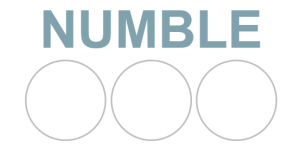Eventually, when the value of each item in the array changes, what’s displayed will also be changed to match.

And within the ‘Keyboard’ `<div>` we have three things:

``````\${state.digits.map((digit,index) => html`<button onclick=\${state.appear(index)}>\${index}</button>`)}
``````

This maps over the array of length 10, creating a button for each item and displaying the `index` of each item. In other words, the digits 0 to 9. Each button also has an inline event listener that calls the event handler `appear` and provides `index` as an argument. However, we will fully explore this in a moment.

Then, we have two buttons, one called ‘Delete’ and the other called ‘Enter’.They both have inline event listeners that call their respective event handlers `remove` and `check`. And again, we will fully explore these in a moment.

Firstly, this is an example of what you’re keyboard could look like: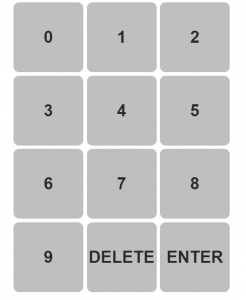Looking at the `appear` event handler, we want this function to display the digit the player clicks into the first space of the `guess`.

``````const appear = guess => event => {
Update(state => ({
guess: state.guess.map((digit,index) => index === state.count ? guess : digit),
count: state.count + 1
}))
}
``````

First of all, the only difference between this event handler and the ones we made before is that this function has an extra parameter `guess`. This is the `index` of the `digits` array that was provided as an argument. In other words, it’s the digit that the player clicked on.

The `Update` function looks a bit different. This is because it needs access to the state, so it is provided with an arrow function that maps the old state to the new state (Nanny State calls these ‘transformer functions’)

In terms of what it’s actually updating, the `guess` property maps over the original array of three `null`s and if the `index` of the item is equal to the `count` (the position of guess), the value of `null` is replaced with the `guess` (which will be the number of the button the user clicked). If the `index` is not equal to `count`, the value of the item is left as it was: `null`.

It then increments `count` by 1, allowing the user to input their second guess into the second space.

This is what the row will look like once the user has clicked a few numbers: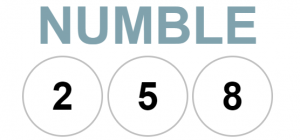The `remove` event handler is (ironically) almost identical:

``````const remove = event => {
Update(state => ({
guess: state.guess.map((digit,index) => index === state.count - 1 ? null : digit),
count: state.count - 1
}))
}
``````

Following the logic of the `appear` function, you should be able to work out what’s going on here, but don’t worry if not. It updates `guess` by mapping over the original array, and if the `index` is equal to the previous number of guesses (i.e. the count – 1), it replaces the value of the item with `null`, effectively deleting the guess.

And this time, it decrements `count` by one, allowing the user to continue to make guesses.

Just the `check` function to go.

The event handler for the ‘Enter’ button is called `check`, and we want this to (surprise) check whether the user’s guess is correct, but we also want it to reset the guess, so the user can try again.

The function looks like this:

``````const check = event => {
Update(state => {
const numble = state.guess.join("") === state.number
return {
feedback: numble ? "NUMBLE!" : "Wrong!",
guess: Array(3).fill(null),
count: 0
}
})
}
``````

Like before, the `Update` uses a transformer function and takes `state` as a parameter, giving us direct access to all the app data held in the state. It then creates a Boolean constant called `numble`. It may not look like it but `state.guess.join("") === state.number` is actually a condition (checks if the user’s guess is equal to the number we generated) and if it meets this condition the value of `numble` will be `true` and if not, it will be `false`.

Then it returns three updated properties of the state:

• `feedback` takes the Boolean value we’ve just created and if it is `true` it sets the value to be the string “NUMBLE!” and if it is `false` it sets the value to be a string “Wrong!”
• `guess` is changed back to an array of length 3 filled with `null`. This will effectively reset the user’s guess, allowing them to guess again
• `count` is also reset to 0, meaning the program can work as if starting from the beginning.

Our final step is to put some HTML in `View` so that feedback can be displayed.

A good place to put it is below the guess and above the keyboard. So, your final `View` should look something like this:

``````const View = state => html`
<h1>Numble</h1>
\${state.started ?
html`<div id="guesses">
\${state.guess.map(number => html`<div>\${number}</div>`)}
</div>
<p id="feedback">\${state.feedback}</p>
<div id="keyboard">
\${state.digits.map((digit,index) => html`<button onclick=\${state.appear(index)}>\${index}</button>`)}
<button onclick=\${state.remove}>DELETE</button>
<button onclick=\${state.check}>ENTER</button>
</div>
<button onclick=\${state.finish}>END</button>`
:
html`<button onclick=\${state.start}>START</button>`
}`
``````

And if you want you can use `feedback` to set a message at the start of the game, for example in the `start` event handler you can add the `feedback` property with a string value (“Guess 3 digits”):

``````const start = event => {
Update({
started: true,
number: generateNumber(),
feedback: "Guess 3 digits"
})
}
``````

And that’s it! You now have a fully functioning guess the number game!

Before you go on to the second article, there are just a couple of notes about CSS and bugs.

If you want to add your own CSS that’s perfectly fine, but if you just want to focus on the code, you can copy my CSS from the final CodePen demo.

If you’re a good programmer you’ll probably be able to pick up on some bugs here, for example, what if the user clicks ‘Enter’ before they’ve guessed three digits? And if you start playing with it, you’ll definitely be able to notice some more.

They aren’t hard to fix at all, you’ll just need a couple of conditions where appropriate. For example, to fix the problem of checking before there are three digits, in the `check` function you can write:

``````const check = event => {
Update(state => {
const numble = state.guess.join("") === state.number
return state.count < 3 ? {
feedback: "too short"
}
:
{
feedback: numble ? "NUMBLE!" : "Wrong!",
guess: Array(3).fill(null),
count: 0
}
})
}
``````

This just checks if the number of guesses is less than 3 and returns different properties with different values accordingly.

We now have a fully functioning ‘Guess the Number’ game and next we will make it more like the full Numble.

## Four Guesses

Our first job is to allow the user 4 guesses. In Wordle, 6 guesses are allowed for a 5-letter word so for Numble we will allow 4 guesses for a three-digit number.

In order to do this we will have to remove the `guess` property and add two more properties to the `State` object:

``````const State = {
started: false,
digits: Array(10).fill("grey"),
guesses: Array(4).fill(Array(3).fill(null)),
guessCount: 0,
count: 0,
start, finish, check, appear, remove,
View
}
``````

As you can see, we now have a `guesses` property in place of the `guess` we had before. The value of `guesses` is a 2D-array, of 4 arrays, each of length three and filled with `null`. If you are unfamiliar with the `Array.fill()` function, it is a shortcut to creating an array meaning we do not have to write out the array in full.

Each of the 4 nested arrays represent one of the 4 guesses the user will make. For example, if the first guess was 123, the `guesses` array would look like this:

``````[[1,2,3], [null, null, null], [null, null, null], [null, null, null]]
``````

Every time the user makes a guess, this array will be updated to match the their guess, effectively keeping a record of all the guesses they have made in the game.

Additionally, we have a `guessCount` property, set with a value of 0. Although similar to the `count` property, it will allow us to keep track of the number of guesses the user has made.

This diagram should help you visualise and fully understand the need for both the `count` and the `guessCount` properties: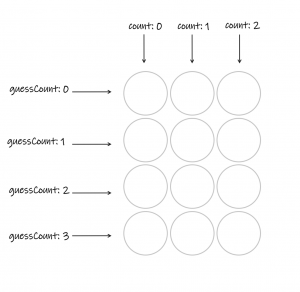As you can see, `guessCount` is the index of which nested array the guess is stored in and `count` is the index of each individual digit of each guess.

Now we need to make some changes to the `View` function:

``````const View = state => html`
<h1>Numble</h1>
\${state.started ?
html`<div id="guesses">
\${state.guesses.map((guess, i) => html`<div class="row">\${guess.map((number,j)=> html`<div class="grey">\${number}</div>`)}</div>`)}
</div>
<p id="feedback">\${state.feedback}</p>
<div id="keyboard">
\${state.digits.map((digit,index) => html`<button onclick=\${state.appear(index)}>\${index}</button>`)}
<button onclick=\${state.remove}>DELETE</button>
<button onclick=\${state.check}>ENTER</button>
</div>
<button onclick=\${state.finish}>END</button>`
:
html`<button onclick=\${state.start}>START</button>`
}`
``````

This is almost identical to the `View` we created earlier, however the div with an id of ‘guesses’ has changed. The fact that we are now using a 2D-array to display the 4 guesses like a grid, we are going to need a nested map.

Coding tip: when using a nested map, for the index of each map we will use `i` for the first map and `j` for the second. You can use whatever you think is easiest for you, so long as they are not the same!

The first map loops over each guess as a row of the grid. The second map then loops over each individual digit of that guess and displays the relevent HTML to show the digit that has been guessed or an empty circle. With this, your screen should look like this: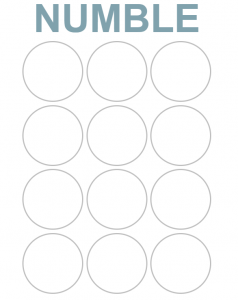This new layout means we also have to change the `appear` and `remove` functions. It is relatively simple but again requires a double map.

``````const appear = guess => event => {
Update(state => ({
guesses:  state.guesses.map((array,i) => i === state.guessCount ? array.map((digit,j) => j === state.count ? guess : digit) : array) ,
count: state.count + 1
}))
}
``````

We are updating the `guesses` property here and this is where having the two different `count` properties is going to become really useful.

The first map checks which row to change: if the index of the array matches to the guess of the user, then the second map can occur, otherwise keep the value the same.

The second map performs the exact same logic as the `appear` we created in article two.

Just like before, the `remove` function works almost identically.

``````const remove = event => {
Update(state => ({
guesses: state.guesses.map((array,i) => i === state.guessCount ? array.map((digit,j)=> j === state.count - 1 ? null : digit) : array),
count: state.count - 1
}))
}
``````

The first map here again just identifies which guess the user is making and the second follows the same logic as our original `remove` function.

The `count` property decrements, however, to ensure the user can re-make the guess.

Finally, we need to make some changes to the `check` function. This is the function that runs every time the user submits a guess.

``````const check = event => {
Update(state => {
const numble = state.guesses[state.guessCount].join("") === state.number
return {
feedback: numble ? "NUMBLE!" : state.guessCount < 3 ? "Keep going..." : `Nope! It was \${state.number}`,
guessCount: state.guessCount + 1,
count: 0
}
})
}
``````

There are only two things that have changed here and both are in the object returned. The `feedback` property has some added logic to make the app a bit more dynamic. The feedback will now display a message to let the user know how they are getting on.

In this case we have: if `numble` is `true` in other words if the user’s guess is correct, the feedback becomes “NUMBLE”; if `numble` is `false`, check if the guess less than three (this essentially checks if the user has made their final guess). If it is, the feedback is “Keep going…”, otherwise it is “Nope! It was (the answer)”.

And that’s it for the first part! You can see the full code in the CodePen demo below.

## Color logic

As outlined at the very beginning of the article, colors are the main focus of Wordle and therefore Numble. If you haven’t already played Numble or Wordle would highly suggest that you do, in order to properly understand the way the colors work.

This is the example of the coloring system, used by Numble: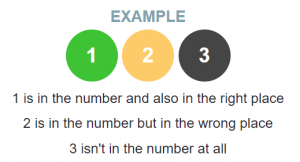After the user makes a guess the colors are updated in two places: the actual guess and on the keyboard. The logic for the two is exactly the same, so we can create an arrow function called `getColors` that takes the guess and the actual number as parameters .

``````const getColors = (guess,number) => guess.map((digit,index) => number.includes(digit) ? digit.toString() === number[index] ? "green" : "yellow": "black")
``````

We map over the ‘guess’ array and using the ‘String.includes(item)’ method, we check first if the answer includes the digit of the guess. If it does, then we check if the digit is in the correct place. If it is, the color is assigned “green”. If not, the color is “yellow”. Otherwise, the digit is not in the answer at all, and so the color is “black”.

This arrow function should then return an array with three items being either “green”, “yellow” or “black”, corresponding to each digit in the ‘guess’.

For example, if we called the function using `getColors([1,2,3], "327")` then the array we should be returned is `["black", "green", "yellow"]`

You may notice we had to change the digit to a string. This is because we need to compare it to the answer that is stored as a string, and you cannot compare two elements if they are of different types. Well you can try, but be prepared to enter a whole world of JavaScript Type coercion pain.

Note: Wordle deals with duplicates differently, so if you wanted to make this a bit harder, you could try and mimic Wordle’s method.

For this next part, we don’t have to make any changes or add anything to `State`, but the `View` does get a bit more complex. As mentioned briefly in the first article, we are going to be using CSS classes to allow us to change the colors.

``````const View = state => html`
<h1>Numble</h1>
\${state.started ?
html`<div id="guesses">
\${state.guesses.map((guess, i) => html`<div class="row">\${guess.map((number,j)=> html`<div class=\${state.guessCount > i ? getColors(guess,state.number)[j] : "grey"}">\${number}</div>`)}</div>`)}
</div>
<p id="feedback">\${state.feedback}</p>
<div id="keyboard">
\${state.digits.map((digit,index) => html`<button class=\${digit} onclick=\${state.appear(index)}>\${index}</button>`)}
<button onclick=\${state.remove}>DELETE</button>
<button onclick=\${state.check}>ENTER</button>
</div>
<button onclick=\${state.finish}>END</button>`
:
html`<button onclick=\${state.start}>START</button>`
}`
``````

As you can see, the only two things that have changed are the CSS classes for both the keyboard buttons and the individual sections of each row.

Beginning with the “guesses” div, we have the following logic:

``````state.guessCount > i ? getColors(guess,state.number)[j] : "grey"
``````

First of all this checks if `guessCount` is higher than the index, this is to ensure that every time the page is rerendered any previous guesses are recolored. If there needs to be a color, we call the `getColors` function with the guess the user has made and the answer as parameters and take the item at the index of each digit, `j`.

Here is what your screen should look like after the user has made one guess: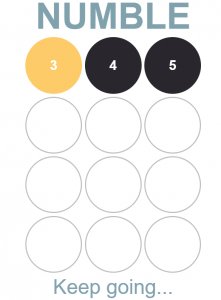The array from the `getColors` function is:

``````["yellow", "black", "black"]
``````

Therefore, the user will now know that 3 is in the number but in the wrong place, and 4 and 5 are not in the number at all.

The keyboard logic is much simpler, but it still uses the same `getColor` function that we wrote before. Remember before how we filled the `digits` array with “grey”? Well this is why we did it.

As the keyboard is drawn on the screen, the class is simply the value at the key’s index in the `digits` array. Later on we will run through how we can change the color, but using the example above after the first guess the `digits` array should look like this:

``````["grey", "grey", "grey", "yellow", "black", "black", "grey", "grey", "grey", "grey"]
``````

We’re very nearly there! Our final job is to change the `check` function.

``````const check = event => {
Update(state => {
const guess = state.guesses[state.guessCount]
const numble = guess.join`` === state.number
const colors = getColors(guess,state.number)
return {
feedback: numble ? "NUMBLE!" : state.guessCount < 3 ? "Keep going..." : `Nope! It was \${state.number}`,
digits: state.digits.map((colour,digit) => guess.includes(digit) ? colors[guess.indexOf(digit)] : colour),
guessCount: state.guessCount + 1,
count: 0
}
})
}
``````

In the `Update` function, there are two more constants. This just makes it easy for the logic in the returned object.

We have `guess` which is the array of three digits the user has just guessed (hence the use of `state.guessCount`). We also have `numble` from before, but this time using the `guess` constant we just created. This just helps having cleaner code and avoiding repetition. Finally, we have `colors` which is the array returned when the `getColors` function is run with the users current guess and the answer.

This will update the digits array and ensure that the digits on the keyboard get colored correctly after each guess.

Now, the return object is identical to the one above but we’re also updating the `digits` property.

``````state.digits.map((color,digit) => guess.includes(digit) ? colors[guess.indexOf(digit)] : color)
``````

This is our final mapping function! And it essentially checks if the number on the keyboard (which is the `digit`) is in the guess. If it is the current color should be replaced by the color generated from the `getColors` function, otherwise the color should remain the same.

Using the same guess as above, we can see what the keyboard should look like: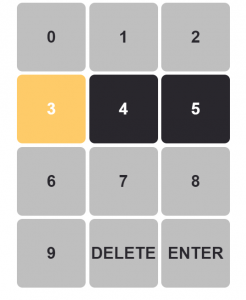And that’s it! A fully functional version of Numble!

Again, here’s what the code should look like in its entirety:

In the actual version of Numble, I added a number of features just to make the game even more dynamic. If you want to challenge yourself and add some extra features here are some suggestions from the my final version of Numble:

• Play again – allow the user to play as many times as they want or make it so there’s only one challenge per day
• Streak – that keeps track of how many correct answers you have in a row
• Best streak – the longest streak the user has kept
• Dark mode – more of a CSS challenge but interesting nontheless
• Display Statistics – a breakdown of how many guesses it took the user for every game
• Share feature – lets users share their best streak

I really hope you had as much fun making Numble as I did!

Original article source at: https://www.sitepoint.com/1671119241

## How to Convert a Number to a String in JavaScript

JavaScript is quite flexible and offers many different ways to convert between data types. In this short tutorial, we’ll look at how you can convert a number to a string in JavaScript. You might want to do this in order to make number data more readable for users — for example, to display the number as part of a sentence.

This tutorial explores four ways to convert a number to a string in JavaScript. We recommend different approaches depending on your specific needs and use case:

• String Interpolation: When inserting a number value within a string. For example, displaying text on a webpage like “You have used 7 credits out of 24”. You can also use Concatenation but beware.
• String or toString(): When changing the type of a number value to a String. For example, using numbers as inputs to functions or APIs that expect a string. `String` and `toString()` are mostly the same but treat `undefined` and `null` variables differently.

You might also be interested to how to convert a string to a number if you’re looking to do the opposite action.

## Convert a Number to a String Using Interpolation

Interpolation is probably the most readable way of using numbers in strings. Instead of manually converting the number to a string, you can insert it into a string using this method.

To use interpolation, wrap a string with backticks (```) instead of quotation marks (`"` or `'`). Then, in the string, you can insert any variable using``\${}`` as a placeholder. This is called a template literal and has a variety of other great benefits.

For example:

``````const number = 99;
console.log(`\${number} percent of people love JavaScript`); // "99% of people love JavaScript"
``````

Since the string that’s being logged into the console is wrapped with backticks, you can insert a variable into the string using `\${}`.

You can see the example in action in the following CodePen demo.

## Convert a Number to a String Using String Concatenation

The second approach is string concatenation. You can convert a number to a string using the `+` operator.

For example:

``````console.log(10 + "USD"); //"10USD"
console.log(10 + ""); //"10"
``````

Although this approach is efficient (as it requires the least amount of code), it can make the code less readable.

### A string concatenation caveat

When using this approach with more than one number, an unexpected result might happen.

For example:

``````const a = 2000;
const b = 468;
console.log(a + b + " motorway"); // "2468 motorway"
``````

Since `a + b` is evaluated first before reaching the string, the operation is a numerical addition rather than a string concatenation. Once a string variable or literal is reached, the operation becomes a string concatenation. So, the result is `2468 motorway`.

However, try changing the code to the following:

``````const a = 2000;
const b = 468;
console.log("it is " + a + b + " motorway"); // "it is 2000468 motorway"
``````

Because `"it is" + a` is evaluated first, the `+` operator is used for string concatenation for the rest of the expression. So, instead of an addition operation between `a` and `b` like the previous example, it becomes a string concatenation operation between the two.

This can be solved using parentheses:

``````const a = 2000;
const b = 468;
console.log("it is " + (a + b) + " motorway"); // "it is 2468 motorway"
``````

The addition between `a` and `b` is performed first, which leads to the addition operation between the two variables. Then, string concatenation is used for the rest of the expression since the first operand is `"it is"`.

## Convert a Number to a String Using toString

The third approach is using the `toString()` method. This method is available for all JavaScript data types, including numbers. It converts the value of the number it’s used on and returns it.

For example:

``````const number = 10;
console.log(number); // 10
console.log(typeof number); // "number"

const numberStr = number.toString();
console.log(numberStr); // "10"
console.log(typeof numberStr); // "string"
``````

This example shows the same result as that of the first approach. You can also see it in action in the following CodePen demo.

## Convert a Number to a String Using String

The fourth approach is using the `String()` constructor function. This function accepts the variable to convert as a first parameter. It converts the parameter to a string and returns it.

For example:

``````const number = 10;
console.log(number); // 10
console.log(typeof number); // "number"

const numberStr = String(number);
console.log(numberStr); // "10"
console.log(typeof numberStr); // "string"
``````

When logging the value of `number` and its type in the console, the result is `10` and `number` respectively. After converting it, the result is `10` as a string and `string` respectively.

You can see the example in action in the following CodePen demo.

## Conclusion

This tutorial shows you four approaches that you can use to convert a number to a string in JavaScript. Although these methods can produce the same results when used with numbers, there are some cases where one approach would be better than the others.

The main difference between using `String()` and `toString()` is that `String()` works with `undefined` and `null` values, whereas `toString()` doesn’t. So, if you have a value that should contain a number but you want to be safe when converting it to a string, you can use `String()`.

As for string interpolation and string concatenation, they’re best used when using numbers within a string. Otherwise, using these methods can make the code less readable.

Original article source at: https://www.sitepoint.com/1671108300

## How to Convert a String to a Number in JavaScript

In JavaScript, there are many ways to convert between data types. We’ve already covered how to convert a number to a string. In this short tutorial, we’ll look at how you can convert a string to a number in JavaScript.

There are many situations where a number might be stored as a string. For example, values received from a form element are always strings.

In general, you can treat a JavaScript string that contains a number (and only a number) as if it were a number, and JavaScript will perform the string-to-number conversion for you automatically. However, sometimes you need to extract a number from a string, or exercise more control over how the conversion is done.

In this quick tip, we’ll cover three ways to convert a string to a number.

## Convert a String to a Number Using Number

The most direct way to convert a string to a number in JavaScript is to use the built-in `Number` constructor function. For example:

``````const str = "10"
console.log(str); // "10"
console.log(typeof str); // "string"

const number = Number(str);
console.log(number); // 10
console.log(typeof number); // "number"
``````

When logging the value of `str` and its type in the console, the result is `10` as a string and `string` respectively. After converting it, the result is `10` as a number and `number` respectively.

You can see the example in action in the following CodePen demo.

Please note that if you pass a non-numeric string to a number, NaN will be returned.

## Convert a String to a Number Using parseInt() and parseFloat()

Another approach is using `parseInt()` and `parseFloat()`. As you can tell, `parseInt()` parses a string to an integer, whereas `parseFloat()` parses a string to a number with decimal points.

For example:

``````const str = "10.9"
console.log(str); // "10.9"
console.log(typeof str); // "string"

const intNumber = parseInt(str);
console.log(intNumber); // 10
console.log(typeof intNumber); // "number"

const floatNumber = parseFloat(str);
console.log(floatNumber); // 10.9
console.log(typeof floatNumber); // "number"
``````

Similar to the first approach, when logging the value of `str` and its type in the console, the result is `10.1` as a string and `string` respectively. However, when parsing `str` using `parseInt`, the value of `intNumber` becomes `10` and its type is `number`.

On the other hand, when parsing `str` using `parseFloat`, the value of `floatNumber` becomes `10.1` as a number and its type is `number`.

You can see the example in action in the following CodePen demo.

### parseInt’s Second Argument

`parseInt()` takes a second argument that specifies the base of the number to be parsed from the string. This argument is in fact optional, but it’s highly recommend that you always provide it.

Without this second argument, `parseInt` performs automatic radix detection. That is, it detects the base of a number by its format in the string. A number beginning `0x` or `0X` is considered to be hexadecimal (base 16), and all other numbers are considered to be decimal.

So, for example, if you were to call `parseInt("08")`, the input value would be considered an octal number; but 8 is not an octal digit (because octal numbering is 0–7), so the function would return a value of zero, not eight.

To avoid any confusion, always specify the base when using `parseInt()`.

## Convert a String to a Number Using Unary Plus

The third way to convert a string to a number is to use the unary plus (`+`). If the unary plus is used before an operand, it attempts to convert it to a number. So, if the operand is a string that contains a number, the unary plus will convert it to a number.

For example:

``````const str = "10";
console.log(typeof str); // "string"
console.log(+str); // 10
console.log(typeof +str); // "number"
``````

When you log the type of `str`, as expected, it’s `string`. However, when you log the value of `+str` and its type, `10` and `number` are logged in the console respectively.

You can see the example in action in the following CodePen demo.

## How to Handle Non-Numeric Characters in Strings

It’s important to take into account cases where a string might contain characters other than numbers and how each approach handles this.

When using `Number()`, if the string contains characters other than numbers, plus(+) and minus(-) signs at the beginning, or decimal points, the returned value is the special value NaN (Not-a-Number). NaN is a global property that represents Not-a-Number. It’s returned by some numerical operations when the operands or parameters of those operations are either not numbers or can’t be used for that specific mathematical operation.

On the other hand, `parseInt()` and `parseFloat()` parse the string if the number is at the beginning of the string. It drops the rest of the characters and takes the number encountered at the beginning of the string. However, if the string starts with characters other than numbers, plus(+) and minus(-) signs at the beginning, or decimal points, the returned value is the special value NaN (Not-a-Number).

You can see it in action in the following CodePen demo.

As you can see, `Number()` returns NaN since the string contains `px`. However, `parseInt()` returns `10` since it’s at the beginning of the string.

You can check if a value is NaN using the global function `isNaN()`.

## Conclusion

This short tutorial has covered three ways to convert a string to a number in JavaScript. `Number()` can convert a string to a number, whether it’s a float or an integer. However, if the string contains other characters, it returns NaN. This is helpful if you want to ensure a strict conversion of the string.

On the other hand, `parseInt()` and `parseFloat()` are more flexible approaches in terms of handling other characters in the number. However, these two functions can’t be used interchangeably in some cases. For example, if the number in the string is a float and you use `parseInt()`, you’ll get an incorrect value compared to the string.

Although the unary is easy to use, it can reduce readability of your code. Whichever method you choose, it’s important to look out for cases where the result returned might be NaN.

Original article source at: https://www.sitepoint.com/1670918160

## Machine Learning Number Recognition

Harnessing the potential of machine learning for computer vision is not a new concept but recent advances and the availability of new tools and datasets have made it more accessible to developers. In this article, Toptal Software Developer Teimur Gasanov demonstrates how you can create an app capable of identifying handwritten digits in under 30 minutes, including the API and UI.

Machine learning, computer vision, building powerful APIs, and creating beautiful UIs are exciting fields witnessing a lot of innovation.

The first two require extensive mathematics and science, while API and UI development center on algorithmic thinking and designing flexible architectures. They are very different, so deciding which one you want to learn next may be challenging. The purpose of this article is to demonstrate how all four can be employed in creating an image processing application.

The application we are going to build is a simple digit recognizer. You draw, the machine predicts the digit. Simplicity is essential because it allows us to see the big picture rather than focus on details.

For the sake of simplicity, we’ll use the most popular and easy-to-learn technologies. The machine learning part will use Python for the back-end application. As for the app’s interactional side, we’ll operate via a JavaScript library that needs no introduction: React.

## Machine Learning to Guess Digits

The core part of our app is the algorithm guessing the drawn number. Machine learning will be the tool used to achieve a good guess quality. This kind of basic artificial intelligence allows a system to learn automatically with a given amount of data. In broader terms, machine learning is a process of finding a coincidence or set of coincidences in the data to rely on them to guess the result.

Our image recognition process contains three steps:

• Get images of drawn digits for training
• Train the system to guess the numbers via training data
• Test the system with new/unknown data

### Environment

We’ll need a virtual environment to work with machine learning in Python. This approach is practical because it manages all the required Python packages, so you don’t need to worry about them.

Let’s install it with the following terminal commands:

``````python3 -m venv virtualenv
source virtualenv/bin/activate
``````

### Training Model

Before we start writing the code, we need to choose a proper “teacher” for our machines. Usually, data science professionals try different models before choosing the best one. We’ll skip very advanced models that require a lot of skill and proceed with the k-nearest neighbors algorithm.

It’s an algorithm that gets some data samples and arranges them on a plane ordered by a given set of characteristics. To understand it better, let’s review the following image:To detect the type of the Green Dot, we should check the types of k nearest neighbors where k is the argument set. Considering the image above, if k is equal to 1, 2, 3, or 4, the guess will be a Black Triangle as most of the green dot’s closest k neighbors are black triangles. If we increase k to 5, then the majority of the objects are blue squares, hence the guess will be a Blue Square.

There are some dependencies needed to create our machine learning model:

• sklearn.neighbors.KNeighborsClassifier is the classifier we’ll use.
• sklearn.model_selection.train_test_split is the function that will help us split the data into training data and data used to check the model’s correctness.
• sklearn.model_selection.cross_val_score is the function to get a mark for the model’s correctness. The higher the value, the better the correctness.
• sklearn.metrics.classification_report is the function to show a statistical report of the model’s guesses.
• sklearn.datasets is the package used to get data for training (images of digits).
• numpy is a package widely used in science as it offers a productive and comfortable way to manipulate multidimensional data structures in Python.
• matplotlib.pyplot is the package used to visualize data.

Let’s start by installing and importing all of them:

``````pip install sklearn numpy matplotlib scipy

from sklearn.neighbors import KNeighborsClassifier
from sklearn.model_selection import train_test_split, cross_val_score
import numpy as np
import matplotlib.pyplot as plt
``````

Now, we need to load the MNIST Database. MNIST is a classic dataset of handwritten images used by thousands of novices in the machine learning field:

``````digits = load_digits()
``````

Once the data is fetched and ready, we can move to the next step of splitting the data into two parts: training and testing.

We’ll use 75% of the data to train our model to guess digits and we shall use the rest of the data to test the model’s correctness:

``````(X_train, X_test, y_train, y_test) = train_test_split(
digits.data, digits.target, test_size=0.25, random_state=42
)
``````

The data is now arranged and we’re ready to use it. We’ll try to find the best parameter k for our model so the guesses will be more precise. We can’t keep the k value off our mind at this stage, as we have to evaluate the model with different k values.

Let’s see why it is essential to consider a range of k values and how this improves our model’s accuracy:

``````ks = np.arange(2, 10)
scores = []
for k in ks:
model = KNeighborsClassifier(n_neighbors=k)
score = cross_val_score(model, X_train, y_train, cv=5)
score.mean()
scores.append(score.mean())

plt.plot(scores, ks)
plt.xlabel('accuracy')
plt.ylabel('k')
plt.show()
``````

Executing this code will show you the following plot describing the algorithm’s accuracy with different k values.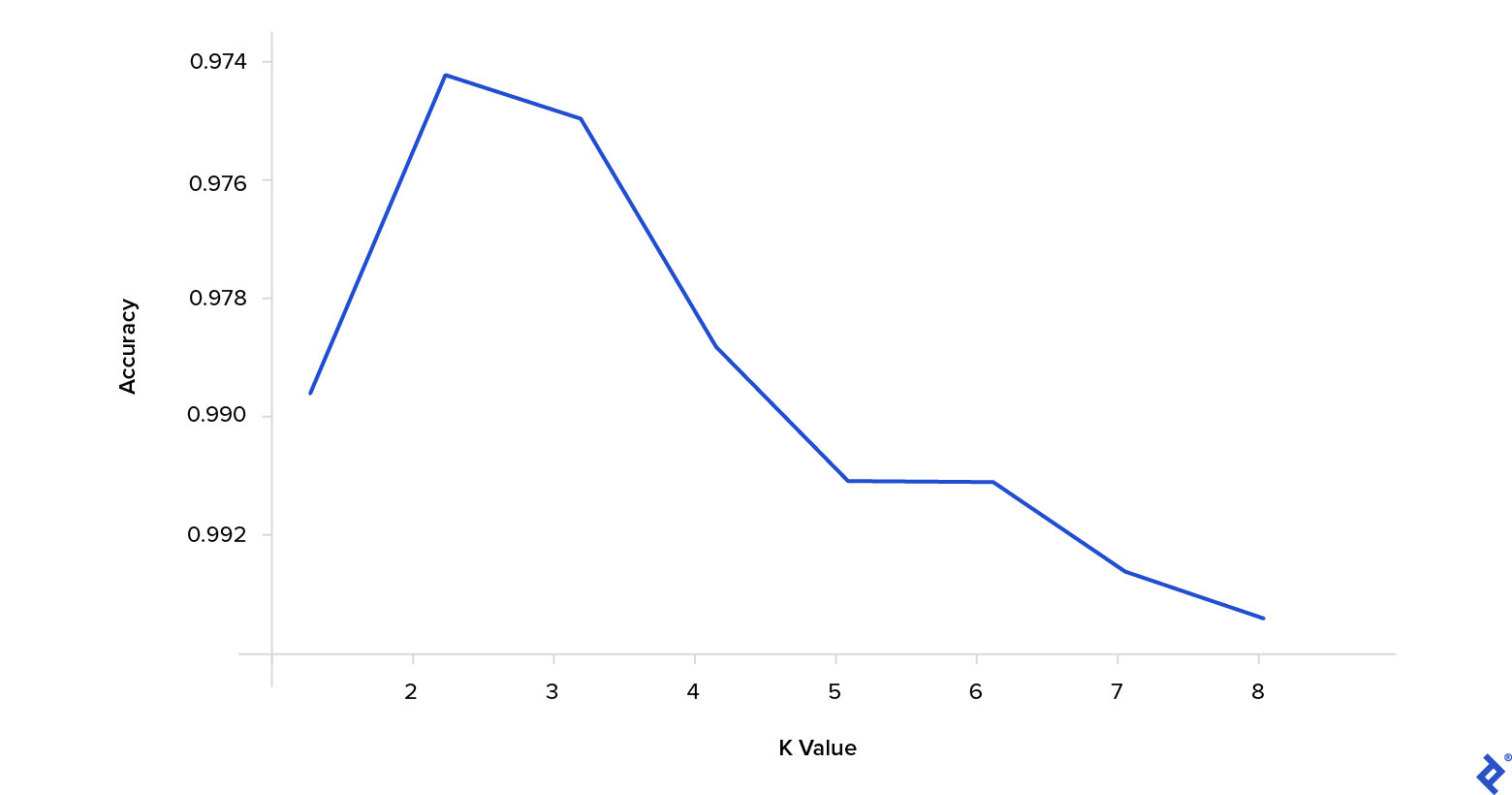As you can see, a k value of 3 ensures the best accuracy for our model and dataset.

## Using Flask to Build an API

The application core, which is an algorithm predicting the digits from images, is now ready. Next, we need to decorate the algorithm with an API layer to make it available for usage. Let’s use the popular Flask web framework to do this cleanly and concisely.

We’ll start by installing Flask and the dependencies related to image processing in the virtual environment:

``````pip install Flask Pillow scikit-image
``````

When the installation completes, we move to the creation of the app’s entry point file:

``````touch app.py
``````

The content of the file will look like this:

``````import os

from views import PredictDigitView, IndexView

'/api/predict',
view_func=PredictDigitView.as_view('predict_digit'),
methods=['POST']
)

'/',
view_func=IndexView.as_view('index'),
methods=['GET']
)

if __name__ == 'main':
port = int(os.environ.get("PORT", 5000))
app.run(host='0.0.0.0', port=port)
``````

You will get an error saying that `PredictDigitView` and `IndexView` are not defined. The next step is creating a file that will initialize these views:

``````from flask import render_template, request, Response

from repo import ClassifierRepo
from services import PredictDigitService
from settings import CLASSIFIER_STORAGE

class IndexView(View):
def dispatch_request(self):
return render_template('index.html')

class PredictDigitView(MethodView):
def post(self):
repo = ClassifierRepo(CLASSIFIER_STORAGE)
service = PredictDigitService(repo)
image_data_uri = request.json['image']
prediction = service.handle(image_data_uri)
return Response(str(prediction).encode(), status=200)
``````

Once again, we will encounter an error about an unresolved import. The Views package relies on three files we do not have yet:

• Settings
• Repo
• Service

We’ll implement them one by one.

Settings is a module with configurations and constant variables. It will store the path to the serialized classifier for us. It begs a logical question: Why do I need to save the classifier?

Because it is a simple way to improve the performance of your app. Instead of training the classifier every time you receive a request, we’ll store the classifier’s prepared version, enabling it to work out of the box:

``````import os

BASE_DIR = os.getcwd()
CLASSIFIER_STORAGE = os.path.join(BASE_DIR, 'storage/classifier.txt')
``````

The mechanism for settings — getting the classifier — will be initialized in the next package on our list, the Repo. It’s a class with two methods to retrieve and update the trained classifier using Python’s built-in `pickle` module:

``````import pickle

class ClassifierRepo:
def __init__(self, storage):
self.storage = storage

def get(self):
with open(self.storage, 'wb') as out:
try:
if classifier_str != '':
else:
return None
except Exception:
return None

def update(self, classifier):
with open(self.storage, 'wb') as in_:
pickle.dump(classifier, in_)
``````

We are close to finalizing our API. Now it lacks only the Service module. What’s its purpose?

• Get the trained classifier from storage
• Transform the image passed from UI to a format the classifier understands
• Calculate the prediction with the formatted image via the classifier
• Return the prediction

Let’s code this algorithm:

``````from sklearn.datasets import load_digits

from classifier import ClassifierFactory
from image_processing import process_image

class PredictDigitService:
def __init__(self, repo):
self.repo = repo

def handle(self, image_data_uri):
classifier = self.repo.get()
if classifier is None:
classifier = ClassifierFactory.create_with_fit(
digits.data,
digits.target
)
self.repo.update(classifier)

x = process_image(image_data_uri)
if x is None:
return 0

prediction = classifier.predict(x)
return prediction
``````

Here you can see that `PredictDigitService` has two dependencies: `ClassifierFactory` and `process_image`.

We’ll start by creating a class to create and train our model:

``````from sklearn.model_selection import train_test_split
from sklearn.neighbors import KNeighborsClassifier

class ClassifierFactory:
@staticmethod
def create_with_fit(data, target):
model = KNeighborsClassifier(n_neighbors=3)
model.fit(data, target)
return model
``````

The API is ready for action. Now we can proceed to the image processing step.

## Image Processing

Image processing is a method of performing certain operations on an image to enhance it or extract some useful information from it. In our case, we need to smoothly transition the image drawn by a user to the machine learning model format.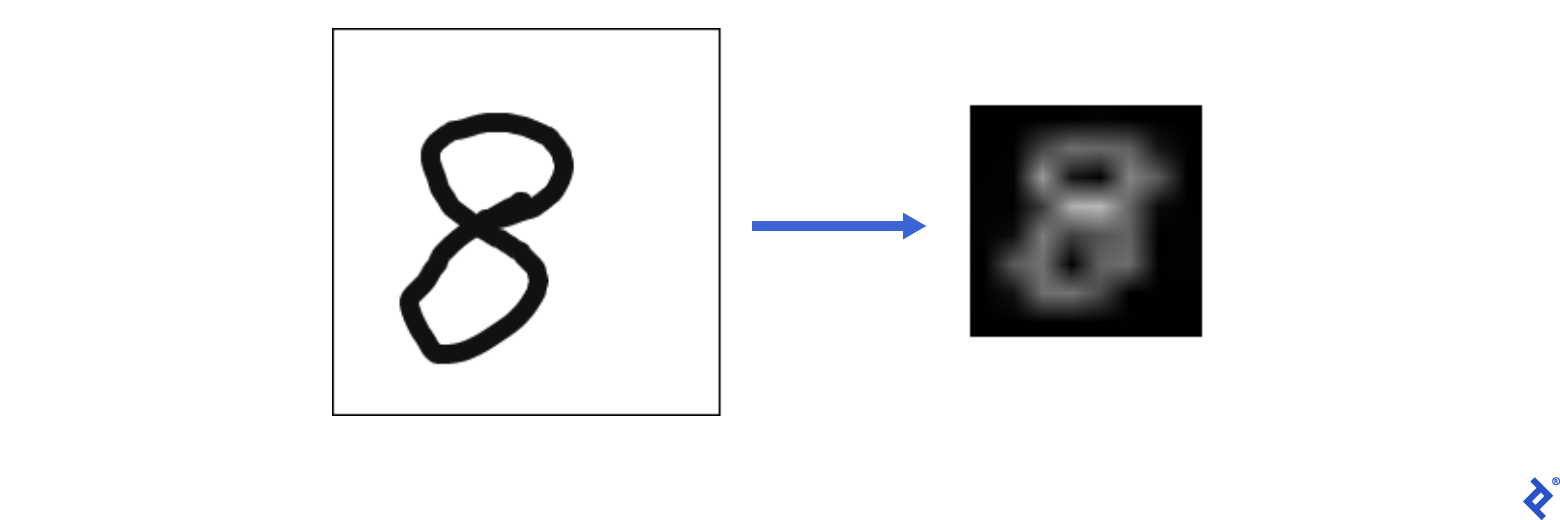Let’s import some helpers to achieve that goal:

``````import numpy as np
from skimage import exposure
import base64
from PIL import Image, ImageOps, ImageChops
from io import BytesIO
``````

We can split the transition into six distinct parts:

1. Replace a transparent background with a color``````def replace_transparent_background(image):
image_arr = np.array(image)

if len(image_arr.shape) == 2:
return image

alpha1 = 0
r2, g2, b2, alpha2 = 255, 255, 255, 255

red, green, blue, alpha = image_arr[:, :, 0], image_arr[:, :, 1], image_arr[:, :, 2], image_arr[:, :, 3]
image_arr[:, :, :4][mask] = [r2, g2, b2, alpha2]

return Image.fromarray(image_arr)
``````

2. Trim open borders``````def trim_borders(image):
bg = Image.new(image.mode, image.size, image.getpixel((0,0)))
diff = ImageChops.difference(image, bg)
diff = ImageChops.add(diff, diff, 2.0, -100)
bbox = diff.getbbox()
if bbox:
return image.crop(bbox)

return image
``````

3. Add borders of equal size``````def pad_image(image):
return ImageOps.expand(image, border=30, fill='#fff')
``````

4. Convert the image to grayscale mode

``````def to_grayscale(image):
return image.convert('L')
``````

5. Invert colors``````def invert_colors(image):
return ImageOps.invert(image)
``````

6. Resize the image to 8x8 format``````def resize_image(image):
return image.resize((8, 8), Image.LINEAR)
``````

Now you can test the app. Run the application and enter the command below to send a request with this iStock image to the API: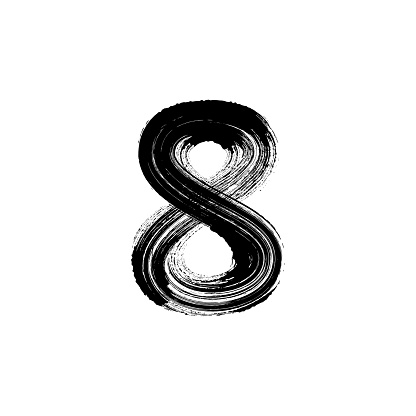``````export FLASK_APP=app
``````
``````curl "http://localhost:5000/api/predict" -X "POST" -H "Content-Type: application/json" -d "{\"image\": \"data:image/png;base64,\$(curl "https://media.istockphoto.com/vectors/number-eight-8-hand-drawn-with-dry-brush-vector-id484207302?k=6&m=484207302&s=170667a&w=0&h=s3YANDyuLS8u2so-uJbMA2uW6fYyyRkabc1a6OTq7iI=" | base64)\"}" -i
``````

You should see the following output:

``````HTTP/1.1 100 Continue

HTTP/1.0 200 OK
Content-Type: text/html; charset=utf-8
Content-Length: 1
Server: Werkzeug/0.14.1 Python/3.6.3
Date: Tue, 27 Mar 2018 07:02:08 GMT

8
``````

The sample image depicted the number 8, and our app correctly identified it as such.

## Creating a Drawing Pane Via React

To quickly bootstrap the frontend application, we’ll use CRA boilerplate:

``````create-react-app frontend
cd frontend
``````

After setting up the workplace, we also need a dependency to draw digits. The react-sketch package matches our needs perfectly:

``````npm i react-sketch
``````

The application has only one component. We can divide this component into two parts: logic and view.

The view part is responsible for representing the drawing pane, Submit and Reset buttons. When interacted, we should also represent a prediction or an error. From the logic perspective, it has the following duties: submit images and clear the sketch.

Whenever a user clicks Submit, the component will extract the image from the sketch component and appeal to the API module’s `makePrediction` function. If the request to the back end succeeds, we’ll set the prediction state variable. Otherwise, we’ll update the error state.

When a user clicks on Reset, the sketch will clear:

``````import React, { useRef, useState } from "react";

import { makePrediction } from "./api";

const App = () => {
const sketchRef = useRef(null);
const [error, setError] = useState();
const [prediction, setPrediction] = useState();

const handleSubmit = () => {
const image = sketchRef.current.toDataURL();

setPrediction(undefined);
setError(undefined);

makePrediction(image).then(setPrediction).catch(setError);
};

const handleClear = (e) => sketchRef.current.clear();

return null
}
``````

The logic is sufficient. Now we can add the visual interface to it:

``````import React, { useRef, useState } from "react";
import { SketchField, Tools } from "react-sketch";

import { makePrediction } from "./api";

import logo from "./logo.svg";
import "./App.css";

const pixels = (count) => `\${count}px`;
const percents = (count) => `\${count}%`;

const MAIN_CONTAINER_WIDTH_PX = 200;
const MAIN_CONTAINER_HEIGHT = 100;
const MAIN_CONTAINER_STYLE = {
width: pixels(MAIN_CONTAINER_WIDTH_PX),
height: percents(MAIN_CONTAINER_HEIGHT),
margin: "0 auto",
};

const SKETCH_CONTAINER_STYLE = {
border: "1px solid black",
width: pixels(MAIN_CONTAINER_WIDTH_PX - 2),
height: pixels(MAIN_CONTAINER_WIDTH_PX - 2),
backgroundColor: "white",
};

const App = () => {
const sketchRef = useRef(null);
const [error, setError] = useState();
const [prediction, setPrediction] = useState();

const handleSubmit = () => {
const image = sketchRef.current.toDataURL();

setPrediction(undefined);
setError(undefined);

makePrediction(image).then(setPrediction).catch(setError);
};

const handleClear = (e) => sketchRef.current.clear();

return (
<div className="App" style={MAIN_CONTAINER_STYLE}>
<div>
<img src={logo} className="App-logo" alt="logo" />
<h1 className="App-title">Draw a digit</h1>
<div style={SKETCH_CONTAINER_STYLE}>
<SketchField
ref={sketchRef}
width="100%"
height="100%"
tool={Tools.Pencil}
imageFormat="jpg"
lineColor="#111"
lineWidth={10}
/>
</div>
{prediction && <h3>Predicted value is: {prediction}</h3>}
<button onClick={handleClear}>Clear</button>
<button onClick={handleSubmit}>Guess the number</button>
{error && <p style={{ color: "red" }}>Something went wrong</p>}
</div>
</div>
);
};

export default App;
``````

The component is ready, test it out by executing and going to `localhost:3000` after:

``````npm run start
``````

The demo application is available here. You can also browse the source code on GitHub.

### Wrapping Up

The quality of this classifier is not perfect, and I do not pretend that it is. The difference between the data we used for training and the data coming from the UI is enormous. Despite that, we created a working application from scratch in less than 30 minutes.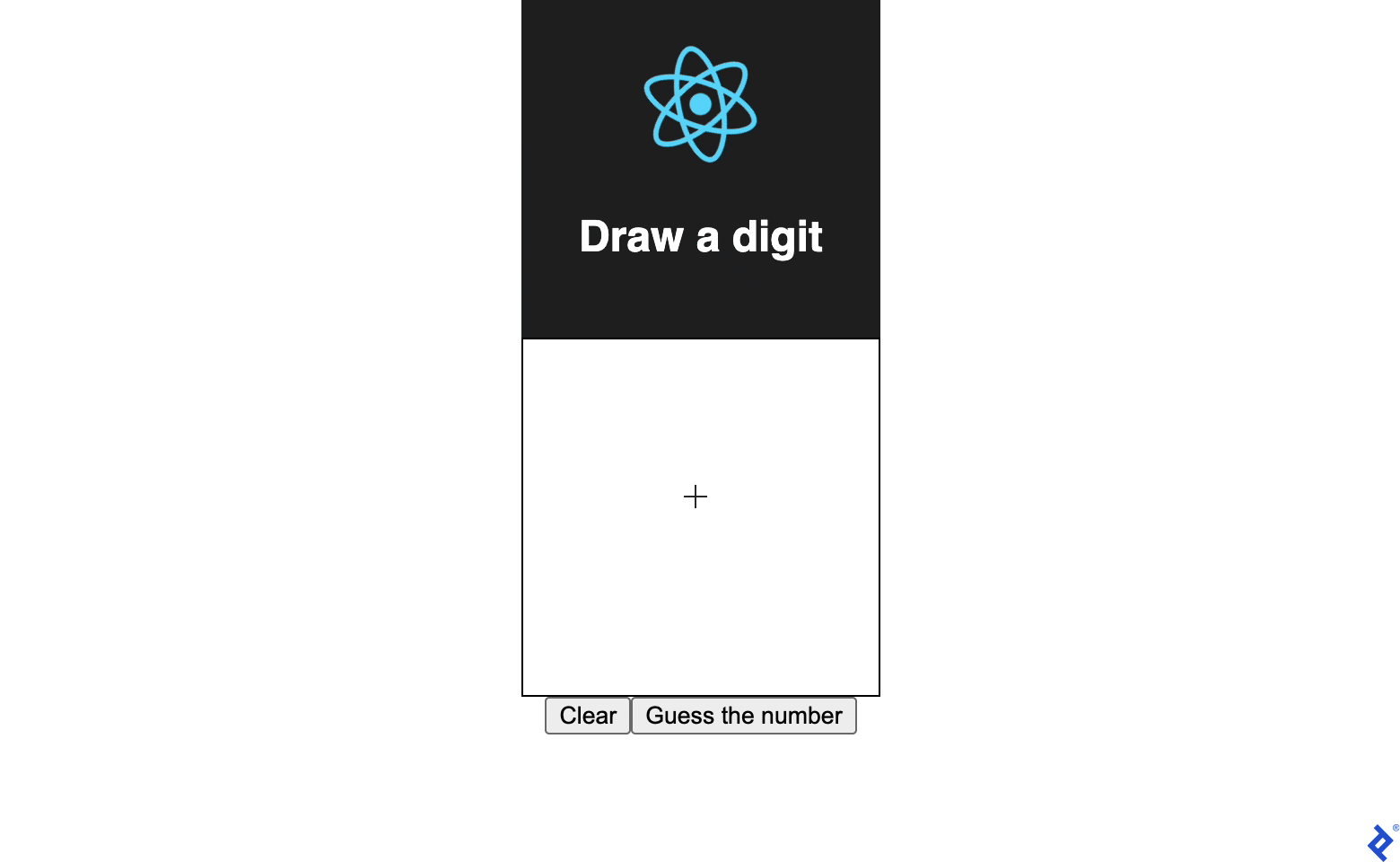In the process, we honed our skills in four fields:

• Machine learning
• Back-end development
• Image processing
• Frontend development

There is no shortage of potential use cases for software capable of recognizing handwritten digits, ranging from education and administrative software to postal and financial services.

Therefore, I hope this article will motivate you to improve your machine learning abilities, image processing, and front-end and back-end development, and use those skills to design wonderful and useful applications.

If you’d like to broaden your knowledge of machine learning and image processing, you may want to check out our Adversarial Machine Learning Tutorial.

Original article source at: https://www.toptal.com/1669705800

## JavaScript format number with commas (example included)

Sometimes, you may need to format a number value with commas in your HTML pages to make it easier to read.

You can transform a number value into a comma-separated string by using JavaScript. Here are two ways to do that:

• Using toLocaleString() method
• Format number with commas using regular expressions
• Conclusion

Let’s learn both ways to format numbers next.

## Using toLocaleString() method

The `Number.toLocaleString()` method is a built-in method of the `Number` object that returns a locale-sensitive string representing the number.

You can pass `"en-US"` as the parameter so that it will always create a thousand separator for your number.

Here’s an example:

``````let n = 234234234;
let str = n.toLocaleString("en-US");
console.log(str); // "234,234,234"
``````

The `toLocaleString()` also works with floating numbers as follows:

``````let n = 234234.555;
let str = n.toLocaleString("en-US");
console.log(str); // "234,234.555"
``````

But if you don’t want to use `toLocaleString()` method, there’s also another way to format numbers with commas.

You can use the `String.replace()` method and regex, which you will learn next.

## Format number with commas using regular expressions

A Regular expression (regex) is a sequence of characters that specifies a search term.

By using regular expressions, you will be able to find and replace values in your string and format them in a way that you require.

For example, consider the following regex pattern:

``````/\B(?=(\d{3})+(?!\d))/g
``````

The regex pattern above will search your string and put a marker when it finds 3 consecutive digits.

You can use the regex pattern in combination with `String.replace()` to replace the markers with commas.

The following example shows how it works:

``````function numberWithCommas(num) {
return num.toString().replace(/\B(?=(\d{3})+(?!\d))/g, ',');
}

let n = numberWithCommas(234234.555);
console.log(n); // "234,234.555"
``````

## Conclusion

And those are two ways you can format a number with commas using JavaScript.

Formatting a number using regex and `replace()` method is always faster than using `toLocaleString()` because `toLocaleString()` method needs to check out the localization (country) selected by your computer or JavaScript engine first before formatting the number.

When you’re handling a huge request to format numbers with commas, it’s probably better to use regex pattern and `String.replace()` method instead of `toLocaleString()` method.

Original article source at: https://sebhastian.com/1669635550

## Get the current year from Date in JavaScript tutorial

When you need to get the current year value from a JavaScript `Date` object, you need to use the `Date.getFullYear()` method, which returns a `number` value representing the year of the date.

For example, the following `Date` object will return `2021` when you call the `getFullYear()` method:

``````const date = new Date("01/20/2021"); // 20th January 2021
const year = date.getFullYear();

console.log(year); // 2021
``````

If you want to get the year represented by 2 digits, you can transform the returned `year` value into a `string` and use the `substring()` method to extract the last 2 digits. Here’s an example:

``````const date = new Date("01/20/2021"); // 20th January 2021
const year = date.getFullYear(); // 2021

const year2digits = year.toString().substring(2);

console.log(year2digits); // "21"
``````

You can cast the 2 digits string representing the year back into number value using the `Number()` method if you need to.

Finally, you can use JavaScript to automatically update an HTML `<footer>` to always show the current year. In the following example, the `<script>` tag will put the `year` value inside the `<span>` tag:

``````<footer>
&copy; <span id="year"></span>
</footer>
<script>
const date = new Date();
const year = date.getFullYear(); // 2021

document.getElementById("year").innerHTML = year;
</script>
``````

And that’s how you can get the current year value using JavaScript.

Original article source at: https://sebhastian.com/1669455487

## JavaScript - Calculating a number's percentage value tutorial

The JavaScript `Math` object doesn’t have any built-in method to calculate the percentage of a number, so if you want to find out the percentage of a specific number, you need to create your own custom function.

For example, the code below will find what 20% of 250 is using the `calcPercent()` function:

``````const percentVal = calcPercent(250, 20);
console.log(percentVal); // 50
``````

To write the `calcPercent()` code, we need to first understand the formula to calculate the percentage number.

To find 20% of 250, you need to divide `20` by 100 and then multiply it by `250`:

``````const num = 250;
const percentage = 20;
const percentVal = 250 * (20 / 100);
console.log(percentVal); // 50
``````

Now that you know the formula for calculating percentages, you can extract the code as a helper function:

``````function calcPercent(num, percentage){
return num * (percentage / 100);
}
``````

Now you can call the helper function `calcPercent()` anytime you need to calculate a number’s percentage value:

``````function calcPercent(num, percentage){
return num * (percentage / 100);
}

let percentVal = calcPercent(250, 20);
console.log(percentVal); // 50
percentVal = calcPercent(210, 10);
console.log(percentVal); // 21
``````

## Rounding the result to 2 decimals

Sometimes, a percentage calculation may return a floating number as follows:

``````const percentVal = calcPercent(20, 3.33);
console.log(percentVal); // 0.666
``````

If you need to round the number to 2 decimals, then you can use a combination of `toFixed()` and `parseFloat()` methods to return the number in 2-decimal format.

Here’s the full function code:

``````function calcPercent(num, percentage){
const result = num * (percentage / 100);
return parseFloat(result.toFixed(2));
}

const percentVal = calcPercent(20, 3.33);
console.log(percentVal); // 0.67
``````

Instead of returning `0.666`, the function will return `0.67` because it rounds the decimal numbers to a maximum length of two.

Original article source at: https://sebhastian.com/1669377060

## JavaScript - how to square a number in three easy ways

Let's learn how to square a number in JavaScript

A square number is a number that you get as a result of multiplying a number by itself. For example, `4` is a square number of `2` because `4 = 2*2`.

This article will show you the three easy ways to square a number using JavaScript. The three different ways you can square a number are as follows:

• Using the `Math.pow()` method
• Using the exponentiation operator (`**`)
• Using a custom helper function `square()`

Let’s begin with using the `Math.pow()` method.

## Square a number using Math.pow() method

The `Math.pow()` method is used to find the exponent value of a number. The exponent value of a number is simply the number itself multiplied as many as X times by an exponent number.

``````Math.pow(number, exponent);
``````

For example, The result of `2` exponent of `4` is `2*2*2*2 = 16`

``````let num = Math.pow(2, 4);
console.log(num); // 16
``````

By using the `Math.pow()` method, you can easily find the square of a number by passing the number `2` as your `exponent` parameter.

Here’s an example of finding the square of the number `6`, which is `36`:

``````let num = Math.pow(6, 2);
console.log(num); // 16
``````

And that’s how you find the square of a number using `Math.pow()` method. Let’s see how you can use the exponentiation operator next.

## Square a number using the exponentiation operator

JavaScript provides you with the exponentiation operator marked by two asterisk symbols (`**`) like this:

``````let num = 2 ** 3 // 2*2*2 = 8
``````

By using the exponentiation operator, you can easily square any number you want.

## Square a number by creating your own helper function

Finally, you can also find the square a number by writing a small helper function as follows:

``````function square(num){
return num * num;
}
``````

By writing a function like `square()` above, you can call the function anytime you need to find the square of a number:

``````console.log(square(2)); // 4
console.log(square(5)); // 25
console.log(square(8)); // 64
``````

This way, you can reduce repetition in your code and make it cleaner.

## Bonus: Finding the square root of a number using JavaScript

Sometimes, you may need to find the square root of a number instead of the square. A square root number is a number that will produce the square number when it’s multiplied by itself:

``````root * root = square;
``````

You can find the square root of a number easily by using the JavaScript `Math.sqrt()` method which accepts the square number as its argument and return the square root number.

Suppose you have the square number of `9`, you can use the `Math.sqrt()` method to find the square root number, which is `3`. Take a look at the code below:

``````let root = Math.sqrt(9);
console.log(root); // 3
``````

Now you’ve learned both how to square a number and how to find the square root of a number using JavaScript. Congratulations! 😉

Original article source at: https://sebhastian.com/1669210863

## How to Create A Floating-point Number using ParseFloat() in JavaScript

JavaScript parseFloat() function explained with examples

The JavaScript `parseFloat()` function is a built-in function that returns a floating-point number from the given `string` argument.

The function allows you to extract a `number` from a `string` as shown below:

``````console.log(parseFloat("12.57.a ")); // 12.57
``````

When a number can’t be parsed from the argument, a `NaN` will be returned by the function:

``````console.log(parseFloat("abc ")); // NaN
``````

The `parseFloat()` function can’t adjust the number of digits for the decimal point.

When you need a certain amount of decimal point digits, you need to use the `toFixed()` method.

For example, suppose you only want 2 decimal point digits out of the `string` number. Here’s how you do it:

``````let string = "37.5930"
let float = parseFloat(string);
let fixedFloatString = float.toFixed(2); // "37.59"
let fixedFloat = parseFloat(fixedFloatString);

console.log(fixedFloat); // 37.59
``````

The `parseFloat()` function is called two times because the `toFixed()` method returns a `string` representing the number where you call the function from.

If you don’t need a `number` type representation of the number, you can omit the last `parseFloat()` function call.

Use isNaN() to check the returned value of parseFloat()

Finally, the `parseFloat()` function returns a `NaN` when the given argument has no valid number representation.

Since a `NaN` isn’t a useful value, you might want to replace it with `0` in some cases.

Consider the following example code:

``````function multiplyByTwo(x) {
if (isNaN(parseFloat(x))) {
x = 0
}
return parseFloat(x) * 2;
}

console.log(multiplyByTwo("abc")) // 0

console.log(multiplyByTwo("3.21`")) // 6.42
``````

When the value of `parseFloat(x)` is `NaN`, the `x` variable will be replaced with `0` instead.

This way, the calculation of `parseFloat(x) * 2` will always return a number `0` in place of `NaN`.

Now you’ve learned how the `parseFloat()` function works in JavaScript. Good work! 😉

Original article source at: https://sebhastian.com/1668859637

Sometimes, you need to add pad a number with leading zeros like numbers displayed in electrical devices.

To add leading zeros to numbers in PHP, you need to format your number as a string using the `sprintf()` function.

Here’s an example of creating a 4 digit number with `sprintf()`:

``````<?php
// 👇 4 digit pad numbers
print sprintf("%04d", 333); // 0333
print PHP_EOL;
print sprintf("%04d", 1234); // 1234
print PHP_EOL;
print sprintf("%04d", 12); // 0012
?>
``````

In the `sprintf()` function above, the format of the string is defined as “format the integer with 4 digits (`4d`) and pad it with leading zeros (`0`)”.

To change the format, you only need to adjust the number before the `d` letter.

The example below shows how to create `6` and `8` digit numbers:

``````<?php

// 👇 6 digit pad numbers
print sprintf("%06d", 1234); // 001234
print PHP_EOL;

// 👇 8 digit pad numbers
print sprintf("%08d", 1234); // 00001234
?>
``````

Because numbers in PHP can’t have leading zeros naturally, you need to format the numbers using the `sprintf()` function.

You can also format negative numbers with leading zeros:

``````<?php
// 👇 6 digit pad numbers
print sprintf("%06d", -123); // -00123
print PHP_EOL;
print sprintf("%06d", -654); // -00654
?>
``````

Alternatively, you can also use the `str_pad()` function to pad numbers with leading zeros.

But this function can’t be used to pad negative numbers as shown below:

``````<?php
// 👇 6 digit pad numbers
print PHP_EOL;

// 👇 8 digit pad numbers
print PHP_EOL;

// 👇 negative number has wrong format
?>
``````

Padding negative numbers with the `str_pad()` function will produce an invalid format.

But if you never need to format negative numbers with leading zeros, then it’s fine to use the `str_pad()` function.

Original article source at: https://sebhastian.com/1668857065

## How to Expr in Bash for Multiplying and Division Of Numbers

The expr command in Bash is used to evaluate expressions. These expressions can take more than 1 argument, which can be anything like regex, integer, string, etc. The expr command in Bash performs basic calculations like addition, subtraction, etc. It also evaluates the string operations like substring, evaluating regular expressions, length of the string, etc. However, many Bash users may get confused in approaches or in multiplying and dividing numbers. Here, we will perform the multiplication and division of numbers in Bash through the expr command.

## Expr in Bash for Multiplication and Division of Numbers

The expr command in Bash reads and evaluates the expression parameters and writes the result to standard output. The syntax of the expr command is:

```expr integer1 operator integer2```

## Multiplication of Numbers in Bash Using the Expr Command

Although “*” symbolizes multiplication, “*” in Bash represents all files in the current directory. If you use “*” directly with the expr for the multiplication of two numbers in the shell, it gives you an error. So, to multiply the numbers in Bash, use “\*’” instead of “*”.

The following example explains how you can multiply the numbers in Bash using the expr command:

``````#! /bin/Bash
#Multiplication of integers using the expr command
A=25
B=5
echo "Multiplication of A and B is (A x B) = AB"
echo "AB= `expr \$A \* \$B`"``````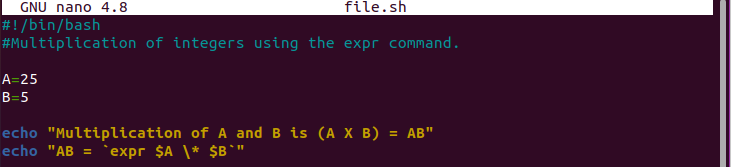Output: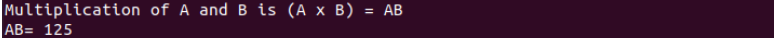## Division of Numbers in Bash Using the Expr Command

Let’s divide the numbers in Bash using the “/” symbol. The following example will give you a better clarification:

``````#! /bin/Bash
#Division of integers using the expr command.
A=25
B=5
echo "A / B = `expr \$A / \$B`"``````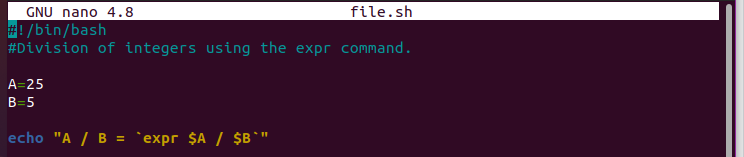Output:## Conclusion

This is how you can multiply and divide the numbers using the expr command in Bash. Creating arithmetic calculations in Bash is simple, and we recommend that you learn these arithmetic operations as beginners. This practice can help you gain a hands-on experience with the Bash scripts. For more information on Bash and shell scripting, please visit the Linuxhint website. We uploaded hundreds of tutorials that are related to Bash and other programming languages.

Original article source at: https://linuxhint.com/1668856339

## Best 9 Ways You Can Convert A String to A Number Type with JavaScript

Learn how you can convert a string to a number type natively using JavaScript

Depending on the conditions you have in your project, there are 9 ways to convert a string type into a number type in JavaScript

When you want a rounded number out of the string, then you can use these functions and methods:

• `parseInt()`
• `Math.round()`
• `Math.floor()`
• `Math.ceil()`

When you want a number with floating points, then you can use these functions and operators:

• `Number()`
• `parseFloat()`
• The unary `+` operator
• The unary `-` operator
• Implicit conversion with the minus, multiplication, division, or remainder operator

Let’s see an example of these functions in action next.

## Create a rounded number from the string

The `parseInt()` function parses a value you passed as its argument and tries to return a number that represents that value.

When you pass a string into the function, it will return a whole number as shown below:

``````parseInt("23"); // 23

// parsing a floating number string:
parseInt("7.31"); // 7

// rounded down even when the floating number > .49
parseInt("7.91"); // 7

// String with no number representative
parseInt("a"); // NaN
``````

Note that strings with floating numbers are always rounded down, even when the floating number is greater than `.49`.

You can use the `Math.round()` method to round the string with floating numbers up or down depending on the floating number’s value:

``````Math.round("2.17"); // 2

// rounded up when floating number >= .5
Math.round("2.5"); // 3

// rounded down when floating number < .5
Math.round("2.49"); // 2
``````

To always round the floating number down, use the `Math.floor()` method:

``````Math.floor("2.17"); // 2

Math.floor("2.5"); // 2

Math.floor("2.49"); // 2
``````

To always round the floating number up, use the `Math.ceil()` method:

``````Math.ceil("2.17"); // 3

Math.ceil("2.5"); // 3

Math.ceil("2.49"); // 3
``````

And that’s how you create a rounded number when converting a string into a number.

Next, let’s learn how to convert a string into a number and preserve the floating points.

## Create a decimal number from the string

Decimal numbers are numbers with floating points. The native `Number()` function in JavaScript will convert your string into its number representation without rounding the floating points:

``````Number("7"); // 7

Number("7.22"); // 7.22

Number("7.52"); // 7.52

Number("7.52.447"); // NaN
``````

Next, the `parseFloat()` function is a native JavaScript function to return a floating-point number from a value.

The code below shows how the `parseFloat()` function works:

``````parseFloat("7"); // 7

parseFloat("7.52"); // 7.52

// still parse number in wrong format:

parseFloat("7.52.447"); // 7.52
parseFloat("7.52aabcs"); // 7.52
``````

When encountering an incorrect number format, the `parseFloat()` function still tries to return a number that represents the string.

On the other hand, the `Number()` function immediately returns a `NaN` when the number format is unrecognized.

The `Number()` function is more strict than the `parseFloat()` function.

Besides these two functions, you can also convert a string of decimal numbers using the unary plus (`+`) and minus (`-`) operator.

The `+` operator evaluates the operand you added next to the operator and tries to return a number as shown below:

``````+"7.22"; // returns 7.22
+"777"; // returns 777

+"-52"; // -52

+"ab"; // NaN
``````

The `-` operator evaluates the given operand and negates the number, creating a negative number in the process:

The code below shows how the `-` operator works:

``````-"7.22"; // -7.22
-"777"; // -777

// negative number string will return a positive number
-"-52"; // 52
-"-52.89"; // 52.89

-"ab"; // NaN
``````

And that’s how the `-` operator can be used to convert a string into a number.

## JavaScript implicit type conversion with minus / multiplication / division / remainder operator

JavaScript automatically converts a string into a number when you use an arithmetic operator except for the unary `+` operator.

Consider the following code examples:

``````"55" - 1; // 54

"5" * 2; // 10

"20" / 5; // 4

"100" % 6; // 4

"-7" - 3; // -10
``````

When you use the `+` operator, JavaScript will concatenate the string and the number instead of converting the string.

In the following code examples, the expressions produce a string instead of a number:

``````// returns a string:

"50" + 1; // "501"
"3.2" + 1; // "32.1"

// returns a number:

Number("5.7") + 3; // 8.7
+"6.1" + 3; // 9.1
``````

You need to manually convert the string into a number before using the `+` operator as shown above.

And that’s how you can convert a string into a number in JavaScript.

Depending on the requirements you have in your project, you can use one of the nine ways mentioned in this tutorial.

Thank you for reading. I hope this tutorial has been useful for you. 🙏

Original article source at: https://sebhastian.com/1668851880

## Format phone numbers in PHP

Learn how you can format phone numbers using PHP

Phone numbers should not be treated as integer numbers because you don’t do math operations on them.

Some phone numbers also start with a zero, and integer type can’t save numbers with a leading zero.

Instead, phone numbers should be saved as strings that are specially formatted as phone numbers.

This tutorial will show you how to format phone numbers in PHP

• Format phone numbers using substr()
• Format phone numbers using regex
• Format phone numbers using libphonenumber

## Format phone numbers using substr()

Formatting phone numbers can be simple or complex depending on the requirements you need to fulfill.

One easy way to format phone numbers in PHP is by calling the `substr()` function to separate your numbers like this:

``````<?php
\$phone_no = "+1735550162";

// 👇 format phone number
\$format_phone =
substr(\$phone_no, -10, -7) . "-" .
substr(\$phone_no, -7, -4) . "-" .
substr(\$phone_no, -4);

print \$format_phone; // 173-555-0162
``````

As you can see, the number `+1735550162` is formatted as `173-555-0162`.

But keep in mind that the `substr()` function can’t handle phone numbers in different formats.

For example, if you have numbers in the US national format like `(111) 234 6789` then the output will be wrong.

To handle different phone numbers format, you need to use a regular expression and `preg_replace()` function.

## Format phone numbers using regex

Here’s an example to format US phone numbers with regex:

``````function format_phone(string \$phone_no) {
return preg_replace(
"/.*(\d{3})[^\d]{0,7}(\d{3})[^\d]{0,7}(\d{4})/",
'(\$1) \$2-\$3',
\$phone_no
);
}

print format_phone("+1-555-549-4137"); // (555) 549-4137
print PHP_EOL;
print format_phone("(555) 096 7286"); // (555) 096-7286
``````

Please keep in mind that the above solution only works for US phone numbers.

Phone numbers from other countries may break the format created using the regular expression above.

Formatting phone numbers is hard because of the different formats and lengths.

If you want to have a complete phone number formatting solution in PHP, you need to write a lot of code for all the possible formats and lengths.

I recommend you use the `libphonenumber` library instead.

## Format phone numbers using libphonenumber

PHP libphonenumber is an implementation of Google’s libphonenumber, a library created for parsing, formatting, and validating phone numbers.

You can install the library using composer, then use the library in your code.

The following example shows how you can parse a phone number from Brazil:

``````<?php

use libphonenumber\NumberParseException;
use libphonenumber\PhoneNumber;
use libphonenumber\PhoneNumberFormat;
use libphonenumber\PhoneNumberUtil;

\$phoneUtil = PhoneNumberUtil::getInstance();

\$phone_no = "+55-955-590-0169";

try {
// 👇 parse the number, pass the country code as second parameter
\$parsed_number = \$phoneUtil->parse(\$phone_no, "BR");

// 👇 get detailed output
print "Input: " . \$phone_no . "\n";
print "Valid number: " .
(\$phoneUtil->isValidNumber(\$parsed_number) ? "true" : "false");
print PHP_EOL;
print "E164: " .
\$phoneUtil->format(\$parsed_number, PhoneNumberFormat::E164);
print PHP_EOL;
print "National: " .
\$phoneUtil->format(\$parsed_number, PhoneNumberFormat::NATIONAL);
print PHP_EOL;
print "International: " .
\$phoneUtil->format(\$parsed_number, PhoneNumberFormat::INTERNATIONAL);
print PHP_EOL;
} catch (NumberParseException \$e) {
var_dump(\$e);
}
``````

The output of the code above will be:

``````Input: +55-955-590-0169
Valid number: true
E164: +559555900169
National: (95) 5590-0169
International: +55 95 5590-0169
``````

Instead of writing your own library, you can use `libphonenumber` to help you format phone numbers with ease.

I hope this tutorial has been useful for you. 🙏

Original article source at: https://sebhastian.com/1668666007

## Expr in Bash for Multiplying and Division Of Numbers

The expr command in Bash is used to evaluate expressions. These expressions can take more than 1 argument, which can be anything like regex, integer, string, etc. The expr command in Bash performs basic calculations like addition, subtraction, etc. It also evaluates the string operations like substring, evaluating regular expressions, length of the string, etc. However, many Bash users may get confused in approaches or in multiplying and dividing numbers. Here, we will perform the multiplication and division of numbers in Bash through the expr command.

## Expr in Bash for Multiplication and Division of Numbers

The expr command in Bash reads and evaluates the expression parameters and writes the result to standard output. The syntax of the expr command is:

```expr integer1 operator integer2```

## Multiplication of Numbers in Bash Using the Expr Command

Although “*” symbolizes multiplication, “*” in Bash represents all files in the current directory. If you use “*” directly with the expr for the multiplication of two numbers in the shell, it gives you an error. So, to multiply the numbers in Bash, use “\*’” instead of “*”.

The following example explains how you can multiply the numbers in Bash using the expr command:

``````#! /bin/Bash
#Multiplication of integers using the expr command
A=25
B=5
echo "Multiplication of A and B is (A x B) = AB"
echo "AB= `expr \$A \* \$B`"``````

Output:

## Division of Numbers in Bash Using the Expr Command

Let’s divide the numbers in Bash using the “/” symbol. The following example will give you a better clarification:

``````#! /bin/Bash
#Division of integers using the expr command.
A=25
B=5
echo "A / B = `expr \$A / \$B`"``````Output:## Conclusion

This is how you can multiply and divide the numbers using the expr command in Bash. Creating arithmetic calculations in Bash is simple, and we recommend that you learn these arithmetic operations as beginners. This practice can help you gain a hands-on experience with the Bash scripts. For more information on Bash and shell scripting, please visit the Linuxhint website. We uploaded hundreds of tutorials that are related to Bash and other programming languages.

Original article source at: https://linuxhint.com/1667425440

## pdf2gerb

Perl script converts PDF files to Gerber format

Pdf2Gerb generates Gerber 274X photoplotting and Excellon drill files from PDFs of a PCB. Up to three PDFs are used: the top copper layer, the bottom copper layer (for 2-sided PCBs), and an optional silk screen layer. The PDFs can be created directly from any PDF drawing software, or a PDF print driver can be used to capture the Print output if the drawing software does not directly support output to PDF.

The general workflow is as follows:

2. Print the top and bottom copper and top silk screen layers to a PDF file.
3. Run Pdf2Gerb on the PDFs to create Gerber and Excellon files.
4. Use a Gerber viewer to double-check the output against the original PCB design.
6. Submit the files to a PCB manufacturer.

Please note that Pdf2Gerb does NOT perform DRC (Design Rule Checks), as these will vary according to individual PCB manufacturer conventions and capabilities. Also note that Pdf2Gerb is not perfect, so the output files must always be checked before submitting them. As of version 1.6, Pdf2Gerb supports most PCB elements, such as round and square pads, round holes, traces, SMD pads, ground planes, no-fill areas, and panelization. However, because it interprets the graphical output of a Print function, there are limitations in what it can recognize (or there may be bugs).

See docs/Pdf2Gerb.pdf for install/setup, config, usage, and other info.

## pdf2gerb_cfg.pm

``````#Pdf2Gerb config settings:
#Put this file in same folder/directory as pdf2gerb.pl itself (global settings),
#or copy to another folder/directory with PDFs if you want PCB-specific settings.
#There is only one user of this file, so we don't need a custom package or namespace.
#NOTE: all constants defined in here will be added to main namespace.
#package pdf2gerb_cfg;

use strict; #trap undef vars (easier debug)
use warnings; #other useful info (easier debug)

##############################################################################################
#configurable settings:
#change values here instead of in main pfg2gerb.pl file

use constant WANT_COLORS => (\$^O !~ m/Win/); #ANSI colors no worky on Windows? this must be set < first DebugPrint() call

#just a little warning; set realistic expectations:
#DebugPrint("\${\(CYAN)}Pdf2Gerb.pl \${\(VERSION)}, \$^O O/S\n\${\(YELLOW)}\${\(BOLD)}\${\(ITALIC)}This is EXPERIMENTAL software.  \nGerber files MAY CONTAIN ERRORS.  Please CHECK them before fabrication!\${\(RESET)}", 0); #if WANT_DEBUG

use constant METRIC => FALSE; #set to TRUE for metric units (only affect final numbers in output files, not internal arithmetic)
use constant APERTURE_LIMIT => 0; #34; #max #apertures to use; generate warnings if too many apertures are used (0 to not check)
use constant DRILL_FMT => '2.4'; #'2.3'; #'2.4' is the default for PCB fab; change to '2.3' for CNC

use constant WANT_DEBUG => 0; #10; #level of debug wanted; higher == more, lower == less, 0 == none
use constant GERBER_DEBUG => 0; #level of debug to include in Gerber file; DON'T USE FOR FABRICATION
use constant WANT_STREAMS => FALSE; #TRUE; #save decompressed streams to files (for debug)
use constant WANT_ALLINPUT => FALSE; #TRUE; #save entire input stream (for debug ONLY)

#DebugPrint(sprintf("\${\(CYAN)}DEBUG: stdout %d, gerber %d, want streams? %d, all input? %d, O/S: \$^O, Perl: \$]\${\(RESET)}\n", WANT_DEBUG, GERBER_DEBUG, WANT_STREAMS, WANT_ALLINPUT), 1);
#DebugPrint(sprintf("max int = %d, min int = %d\n", MAXINT, MININT), 1);

#define standard trace and pad sizes to reduce scaling or PDF rendering errors:
#This avoids weird aperture settings and replaces them with more standardized values.
#(I'm not sure how photoplotters handle strange sizes).
#Fewer choices here gives more accurate mapping in the final Gerber files.
#units are in inches
use constant TOOL_SIZES => #add more as desired
(
#round or square pads (> 0) and drills (< 0):
.010, -.001,  #tiny pads for SMD; dummy drill size (too small for practical use, but needed so StandardTool will use this entry)
.031, -.014,  #used for vias
.041, -.020,  #smallest non-filled plated hole
.051, -.025,
.056, -.029,  #useful for IC pins
.070, -.033,
#    .090, -.043,  #NOTE: 600 dpi is not high enough resolution to reliably distinguish between .043" and .046", so choose 1 of the 2 here
.100, -.046,
.115, -.052,
.130, -.061,
.140, -.067,
.150, -.079,
.175, -.088,
.190, -.093,
.200, -.100,
.220, -.110,
.160, -.125,  #useful for mounting holes
#some additional pad sizes without holes (repeat a previous hole size if you just want the pad size):
.090, -.040,  #want a .090 pad option, but use dummy hole size
.065, -.040, #.065 x .065 rect pad
.035, -.040, #.035 x .065 rect pad
#traces:
.001,  #too thin for real traces; use only for board outlines
.006,  #minimum real trace width; mainly used for text
.008,  #mainly used for mid-sized text, not traces
.010,  #minimum recommended trace width for low-current signals
.012,
.015,  #moderate low-voltage current
.020,  #heavier trace for power, ground (even if a lighter one is adequate)
.025,
.030,  #heavy-current traces; be careful with these ones!
.040,
.050,
.060,
.080,
.100,
.120,
);
#Areas larger than the values below will be filled with parallel lines:
#This cuts down on the number of aperture sizes used.
#Set to 0 to always use an aperture or drill, regardless of size.
use constant { MAX_APERTURE => max((TOOL_SIZES)) + .004, MAX_DRILL => -min((TOOL_SIZES)) + .004 }; #max aperture and drill sizes (plus a little tolerance)
#DebugPrint(sprintf("using %d standard tool sizes: %s, max aper %.3f, max drill %.3f\n", scalar((TOOL_SIZES)), join(", ", (TOOL_SIZES)), MAX_APERTURE, MAX_DRILL), 1);

#NOTE: Compare the PDF to the original CAD file to check the accuracy of the PDF rendering and parsing!
#for example, the CAD software I used generated the following circles for holes:
#CAD hole size:   parsed PDF diameter:      error:
#  .014                .016                +.002
#  .020                .02267              +.00267
#  .025                .026                +.001
#  .029                .03167              +.00267
#  .033                .036                +.003
#  .040                .04267              +.00267
#This was usually ~ .002" - .003" too big compared to the hole as displayed in the CAD software.
#To compensate for PDF rendering errors (either during CAD Print function or PDF parsing logic), adjust the values below as needed.
#units are pixels; for example, a value of 2.4 at 600 dpi = .0004 inch, 2 at 600 dpi = .0033"
use constant
{
HOLE_ADJUST => -0.004 * 600, #-2.6, #holes seemed to be slightly oversized (by .002" - .004"), so shrink them a little
RNDPAD_ADJUST => -0.003 * 600, #-2, #-2.4, #round pads seemed to be slightly oversized, so shrink them a little
SQRPAD_ADJUST => +0.001 * 600, #+.5, #square pads are sometimes too small by .00067, so bump them up a little
TRACE_ADJUST => 0, #(pixels) traces seemed to be okay?
REDUCE_TOLERANCE => .001, #(inches) allow this much variation when reducing circles and rects
};

#Also, my CAD's Print function or the PDF print driver I used was a little off for circles, so define some additional adjustment values here:
#Values are added to X/Y coordinates; units are pixels; for example, a value of 1 at 600 dpi would be ~= .002 inch
use constant
{
CIRCLE_ADJUST_MINY => -0.001 * 600, #-1, #circles were a little too high, so nudge them a little lower
CIRCLE_ADJUST_MAXX => +0.001 * 600, #+1, #circles were a little too far to the left, so nudge them a little to the right
SUBST_CIRCLE_CLIPRECT => FALSE, #generate circle and substitute for clip rects (to compensate for the way some CAD software draws circles)
WANT_CLIPRECT => TRUE, #FALSE, #AI doesn't need clip rect at all? should be on normally?
RECT_COMPLETION => FALSE, #TRUE, #fill in 4th side of rect when 3 sides found
};

use constant SOLDER_MARGIN => +.012; #units are inches

#line join/cap styles:
use constant
{
CAP_NONE => 0, #butt (none); line is exact length
CAP_ROUND => 1, #round cap/join; line overhangs by a semi-circle at either end
CAP_SQUARE => 2, #square cap/join; line overhangs by a half square on either end
CAP_OVERRIDE => FALSE, #cap style overrides drawing logic
};

#number of elements in each shape type:
use constant
{
RECT_SHAPELEN => 6, #x0, y0, x1, y1, count, "rect" (start, end corners)
LINE_SHAPELEN => 6, #x0, y0, x1, y1, count, "line" (line seg)
CURVE_SHAPELEN => 10, #xstart, ystart, x0, y0, x1, y1, xend, yend, count, "curve" (bezier 2 points)
CIRCLE_SHAPELEN => 5, #x, y, 5, count, "circle" (center + radius)
};
#const my %SHAPELEN =
our %SHAPELEN =
(
rect => RECT_SHAPELEN,
line => LINE_SHAPELEN,
curve => CURVE_SHAPELEN,
circle => CIRCLE_SHAPELEN,
);

#panelization:
#This will repeat the entire body the number of times indicated along the X or Y axes (files grow accordingly).
#Display elements that overhang PCB boundary can be squashed or left as-is (typically text or other silk screen markings).
#Set "overhangs" TRUE to allow overhangs, FALSE to truncate them.
use constant PANELIZE => {'x' => 1, 'y' => 1, 'xpad' => 0, 'ypad' => 0, 'overhangs' => TRUE}; #number of times to repeat in X and Y directions

# Set this to 1 if you need TurboCAD support.
#\$turboCAD = FALSE; #is this still needed as an option?

#CIRCAD pad generation uses an appropriate aperture, then moves it (stroke) "a little" - we use this to find pads and distinguish them from PCB holes.
use constant PAD_STROKE => 0.3; #0.0005 * 600; #units are pixels
#convert very short traces to pads or holes:
use constant TRACE_MINLEN => .001; #units are inches
#use constant ALWAYS_XY => TRUE; #FALSE; #force XY even if X or Y doesn't change; NOTE: needs to be TRUE for all pads to show in FlatCAM and ViewPlot
use constant REMOVE_POLARITY => FALSE; #TRUE; #set to remove subtractive (negative) polarity; NOTE: must be FALSE for ground planes

#PDF uses "points", each point = 1/72 inch
#combined with a PDF scale factor of .12, this gives 600 dpi resolution (1/72 * .12 = 600 dpi)
use constant INCHES_PER_POINT => 1/72; #0.0138888889; #multiply point-size by this to get inches

# The precision used when computing a bezier curve. Higher numbers are more precise but slower (and generate larger files).
#\$bezierPrecision = 100;
use constant BEZIER_PRECISION => 36; #100; #use const; reduced for faster rendering (mainly used for silk screen and thermal pads)

# Ground planes and silk screen or larger copper rectangles or circles are filled line-by-line using this resolution.
use constant FILL_WIDTH => .01; #fill at most 0.01 inch at a time

# The max number of characters to read into memory
use constant MAX_BYTES => 10 * M; #bumped up to 10 MB, use const

use constant DUP_DRILL1 => TRUE; #FALSE; #kludge: ViewPlot doesn't load drill files that are too small so duplicate first tool

my \$runtime = time(); #Time::HiRes::gettimeofday(); #measure my execution time

print STDERR "Loaded config settings from '\${\(__FILE__)}'.\n";
1; #last value must be truthful to indicate successful load

#############################################################################################
#junk/experiment:

#use Package::Constants;
#use Exporter qw(import); #https://perldoc.perl.org/Exporter.html

#my \$caller = "pdf2gerb::";

#sub cfg
#{
#    my \$proto = shift;
#    my \$class = ref(\$proto) || \$proto;
#    my \$settings =
#    {
#        \$WANT_DEBUG => 990, #10; #level of debug wanted; higher == more, lower == less, 0 == none
#    };
#    bless(\$settings, \$class);
#    return \$settings;
#}

#use constant HELLO => "hi there2"; #"main::HELLO" => "hi there";
#use constant GOODBYE => 14; #"main::GOODBYE" => 12;

#our @EXPORT_OK = Package::Constants->list(__PACKAGE__); #https://www.perlmonks.org/?node_id=1072691; NOTE: "_OK" skips short/common names

#print STDERR scalar(@EXPORT_OK) . " consts exported:\n";
#foreach(@EXPORT_OK) { print STDERR "\$_\n"; }
#my \$val = main::thing("xyz");
#print STDERR "caller gave me \$val\n";
#foreach my \$arg (@ARGV) { print STDERR "arg \$arg\n"; }``````